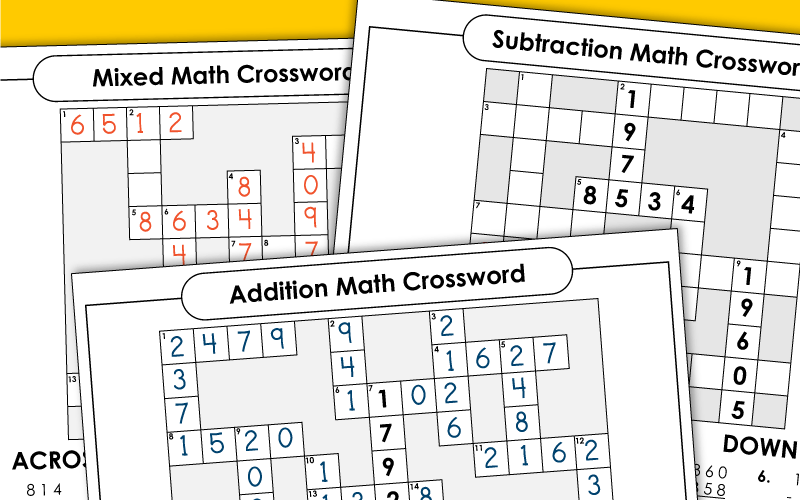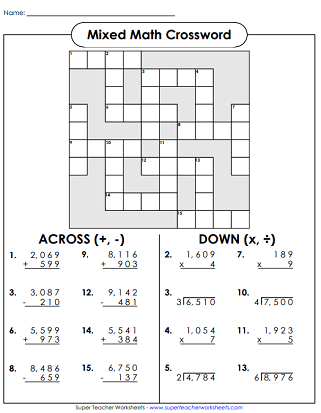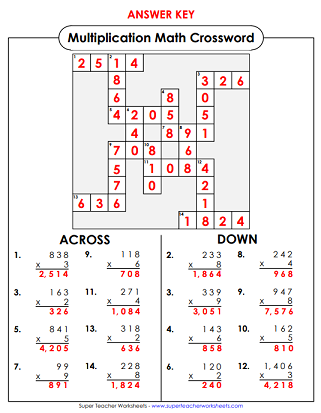# Math Crosswords

Math crossword puzzles make it fun for students to review addition, subtraction, multiplication, and division.## Subtraction

Find the answers to the subtraction problems and complete the number crossword puzzle.
Subtract to find th four and five digit differences.  The plug the numbers into the puzzle.

## Multiplication

Multiply the numbers to find the products.  Use the answers to finish the crossword puzzle.
Multiply to find the products and place the answers in the puzzle.
Find the products of these four-digit by two-digit multiplication problems.  Then complete the puzzle.

## Division

Divide the four digit dividends.  Use the quotients in the puzzle.

## Mixed

Add, subtract, divide, and multiply to solve this math crossword.  (Grade 4 and up)

Math Mystery Pictures

To complete the mystery pictures, students must practice addition, subtraction, multiplication, or division skills.

## Sample Worksheet ImagesMy Account
Site Information